• Posts tagged "区间抽样"

# Blog Archives

## 抽样三步曲之二：R语言进行抽样估计

• 张丹, 分析师/程序员/Quant: R,Java,Nodejs
• blog: http://blog.fens.me
• email: bsspirit@gmail.com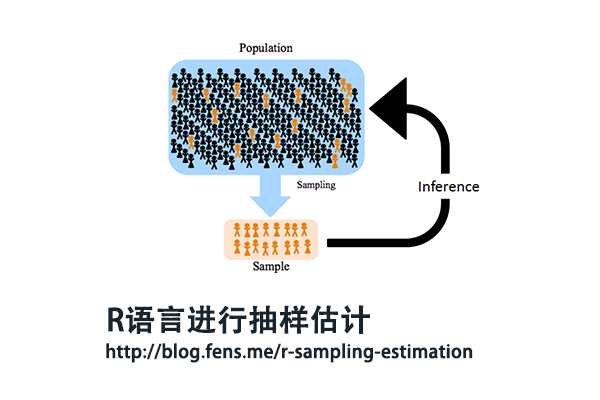1. 抽样估计介绍
2. 点估计
3. 抽样误差
4. 区间估计

## 1. 抽样估计介绍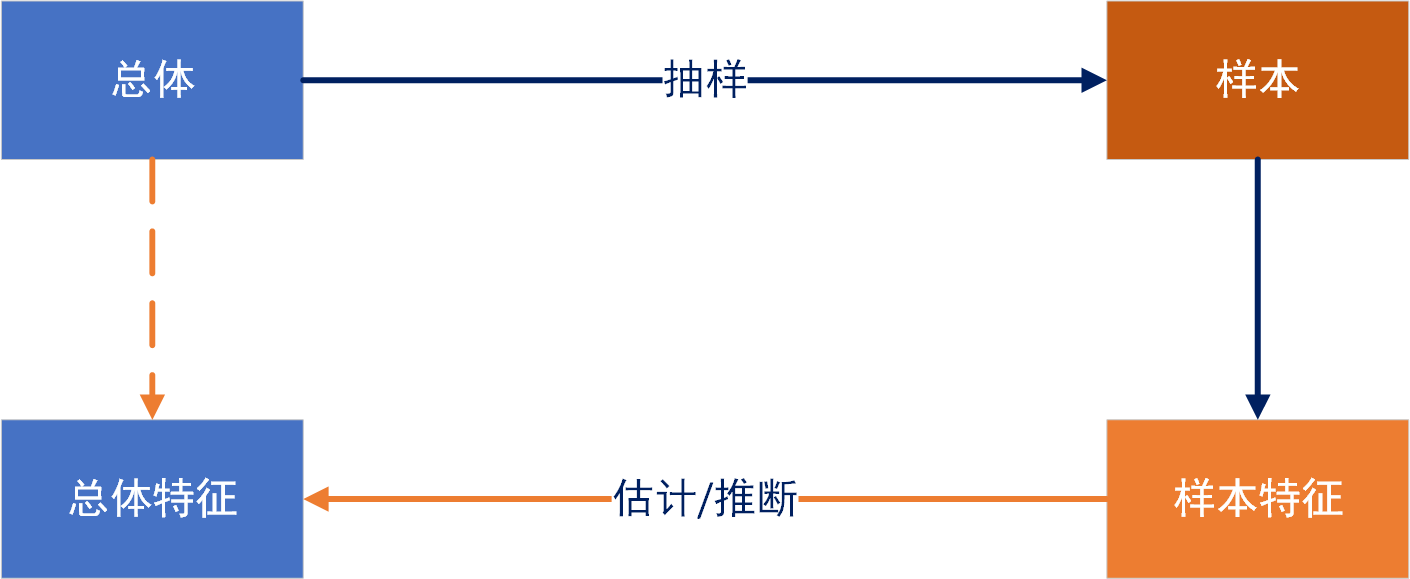## 2. 点估计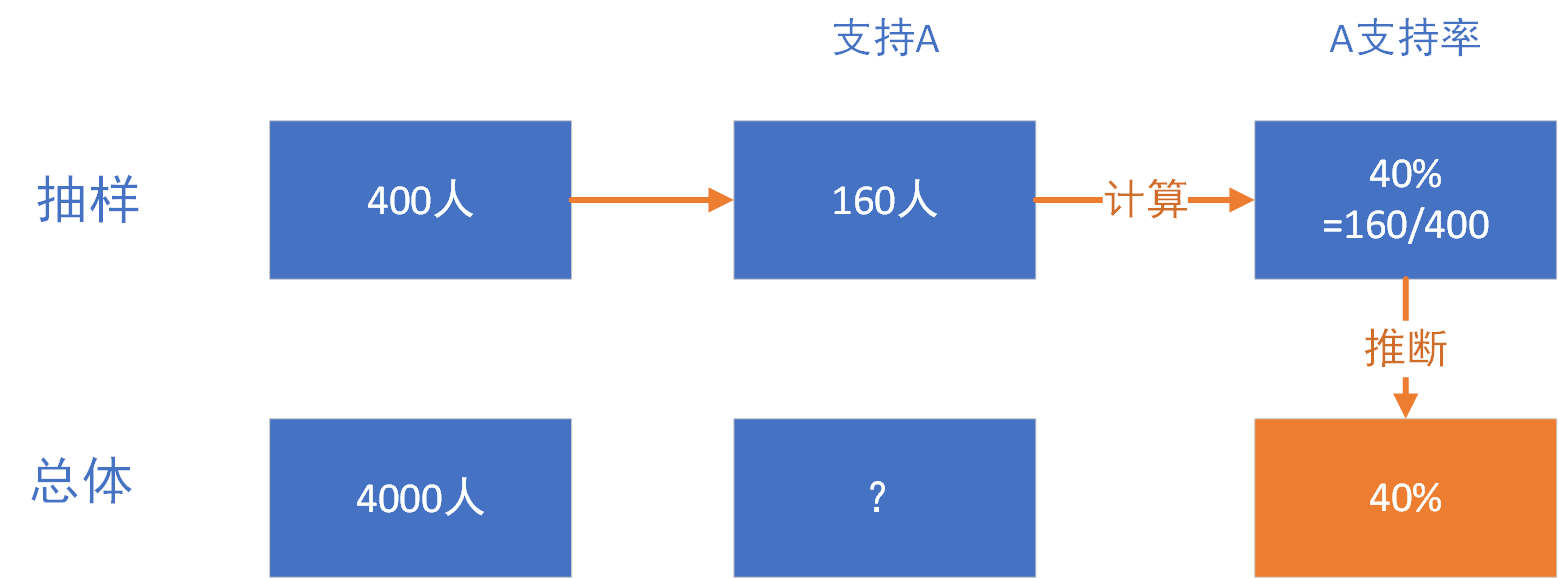• 无偏性。即要求所有可能样本指标的平均数（样本指标的数学期望）与被估计的总体参数之间没有偏差。虽然每一次的样本指标值和总体指标值之间都可能有误差，但在多次反复的估计中，所有抽样指标值的平均数应该等于所估计的总体指标值本身，即用样本指标去估计总体参数，平均说来是没有偏误的。
• 一致性。用统计量估计总体参数要求当样本的单位数充分大时，抽样指标也充分地靠近总体指标。就是说，随着样本单位数n的无限增加，统计量和未知的总体参数之差的绝对值小于任意小的数，它的概率也趋近于1，即实际上是几乎肯定的。
• 有效性。以统计量估计总体参数时，优良估计量的方差应该比其他估计量的方差小。例如用样本平均数或总体某一变量值来估计总体平均数，虽然两者都是无偏的，而且在每一次估计中，两种估计量和总体平均数都可能有离差，但样本平均数更靠近于总体平均数的周围，平均说来其离差比较小。所以对比说来，抽样平均数是更为有效的估计量。

1. 生成总体数据
2. 抽样获得样本，并计算样本特征
3. 多次抽样
4. 抽样分布
5. 获得结论

1. 生成总体数据

# N总体4000人
> N<-4000

# 工资，使用均匀分布和正态分布进行叠加
> set.seed(0)
> salary1<-runif(2000,30000,80000)  # 均匀分布
> set.seed(0)
> salary2<-rnorm(2000,51814,3000)   # 正态分布
> salary<-c(salary1,salary2)

# 20个地区
> set.seed(1)                 #设置随机种子
> area<-sample(1:20,N,TRUE)

# 投票，1为投票给A，0为不投票给A
> set.seed(2)                 #设置随机种子
> vote<-sample(1:N,1500)

# 总体数据集
> df<-data.frame(salary,area)
> df$area<-as.factor(df$area)
> df$vote<-0 > df$vote[vote]<-1
> df$vote<-as.factor(df$vote)

# 查看数据前10条
salary area vote
1  74834.86    4    0
2  43275.43    7    0
3  48606.19    1    1
4  58642.67    2    0
5  75410.39   11    0
6  40084.10   14    0
7  74919.48   18    0
8  77233.76   19    1
9  63039.89    1    0
10 61455.70   10    1

# 查看统计概率
> summary(df)
salary           area      vote
Min.   :30030   13     : 226   0:2500
1st Qu.:48302   20     : 217   1:1500
Median :52015   9      : 215
Mean   :53254   14     : 215
3rd Qu.:56654   8      : 211
Max.   :79997   6      : 206
(Other):2710


• salary，每个选民的工资
• area，每个选民所在地区，1到20
• vote，对A的投票

2. 抽样获得样本，并计算样本特征

# 加载工具包
> library(sampling)
> library(plyr)
> library(magrittr)

# 抽样400人
> s<-400
> set.seed(30)             # 随机种子
> s1<-srswor(s,N)      # 简单随机抽样

# 样本数据集
> sample_df<-getdata(df,s1)

# 样本工资均值
> mean(sample_df$salary)  53936.74 # 样本工资标准差 > sd(sample_df$salary)
 10930.51

# 样本工资方差
> var(sample_df$salary)  119476082 # 样本投票比例 > table(sample_df$vote)
0   1
242 158

# 地区分布
> table(sample_df$area) 1 2 3 4 5 6 7 8 9 10 11 12 13 14 15 16 17 18 19 20 12 21 24 17 22 17 20 25 19 17 20 15 24 24 18 22 20 23 26 14  对选样本选民资进行正态分布检验。  > shapiro.test(sample_df$salary)
Shapiro-Wilk normality test
data:  sample_df$salary W = 0.94848, p-value = 1.389e-10 # 画出qq图 > qqnorm(sample_df$salary)
> qqline(sample_df$salary,col="red")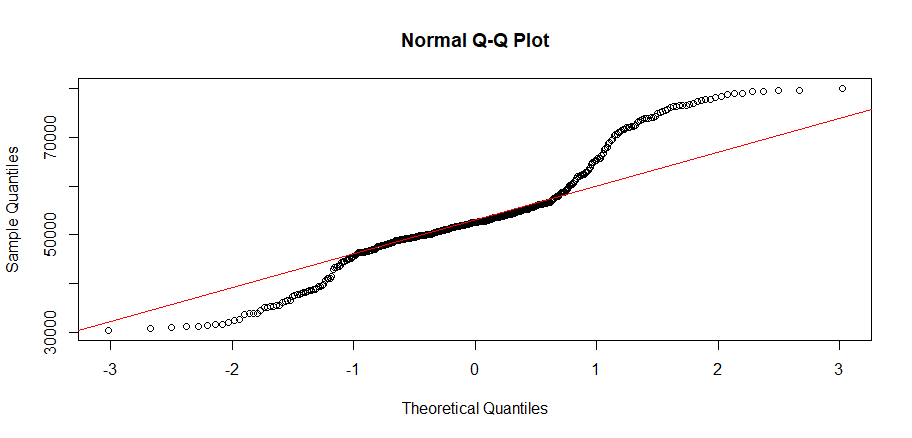由于p-value=0.1389 > 0.05，不能拒绝正在分布的假设，所以，工资符合正态分布。但从图中看，已经偏离很大了。这样我们就完成一次抽样的过程了。 3. 多次抽样 1次抽样不能说明什么问题，我们进行多次抽样，进行500次随机抽样，可能得到不同的结果，形成不同的抽样分布。  # 定义抽样数据框 > sample500<-data.frame(time=1:500,mean=0,var=0,vote=0) # 进行500次随机抽样 > for(i in 1:500){ + s1<-srswor(s,N) + sample_df<-getdata(df,s1) + sample500$mean[i]<-mean(sample_df$salary) + sample500$var[i]<-var(sample_df$salary) + sample500$vote[i]<-length(which(sample_df$vote==1))/s + } # 查看前6条数据 > head(sample500) time mean var vote 1 1 53457.14 118965345 0.4000 2 2 53138.77 98917316 0.4150 3 3 52852.20 113358728 0.3925 4 4 53655.00 104086558 0.3525 5 5 52530.54 107175129 0.3625 6 6 53491.33 119491300 0.3700  这时，我们完成了500次的抽样，并把抽样的结果进行了整理。 4. 抽样分布 为了观察方例，以柱状图进行可视化，查看每个统计量的抽样分布。  # 图片输出布局，一行三列 > par(mfrow=c(1,3)) # 输出样本均值，样本方差，样本投票率的柱状图 hist(sample500$mean,10,ylim=c(0,200),main='mean')
hist(sample500$var,10,ylim=c(0,200),main='var') hist(sample500$vote,10,ylim=c(0,200),main='vote')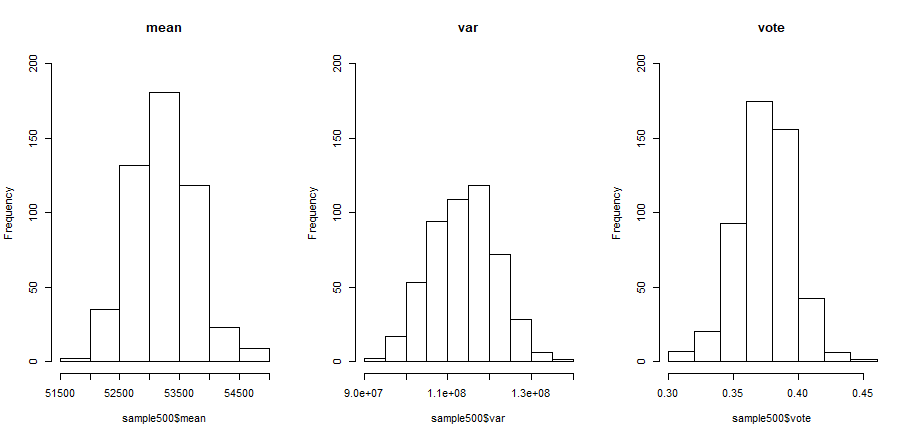# 均值的正态分布检查
> shapiro.test(sample500$mean) Shapiro-Wilk normality test data: sample500$mean
W = 0.99745, p-value = 0.6438

# 方差的正态分布检查
> shapiro.test(sample500$var) Shapiro-Wilk normality test data: sample500$var
W = 0.99503, p-value = 0.1084

# 投票率的正态分布检查
> shapiro.test(sample500$vote) Shapiro-Wilk normality test data: sample500$vote
W = 0.99465, p-value = 0.07877


5. 获得结论

> colMeans(sample500[,c("mean","var","vote")])
mean          var         vote
5.323558e+04 1.136433e+08 3.751550e-01


## 3. 抽样误差

 数据集 数量 salary均值 vote投票支持率 总体 4000 53253.55 37.5% 1次抽样 400 54170.98 39.5% 500次抽样 400*500 53235.58 37.52%


N为总体数量，s为样本数量

sd_sample = sqrt((N-s)/(N-1)) * （sd_total / sqrt(s)）

sd_sample = sd_total / sqrt(s)




> N<- 4000
> s<- 400

# 在有限个总体的情况下，修正因子
> fpc<-sqrt((N-s)/(N-1)) ;fpc
 0.9488019

# 均值标准误
> fpc * sd(df$salary) / sqrt(sd(sample500$mean))
 447.9483



# 总体均值为红色线
> total_mean<-mean(df$salary) > par(mfrow=c(1,4)) > hist(sample500$mean[1:30],breaks=20,xlim=c(51000,55000),ylim=c(0,80),main="30")
> abline(v = total_mean,col="red")
> hist(sample500$mean[1:100],breaks=20,xlim=c(51000,55000),ylim=c(0,80),main="100") > abline(v =total_mean,col="red") > hist(sample500$mean[1:200],breaks=20,xlim=c(51000,55000),ylim=c(0,80),main="200")
> abline(v =total_mean,col="red")
> hist(sample500\$mean[1:500],breaks=20,xlim=c(51000,55000),ylim=c(0,80),main="500")
> abline(v =total_mean,col="red")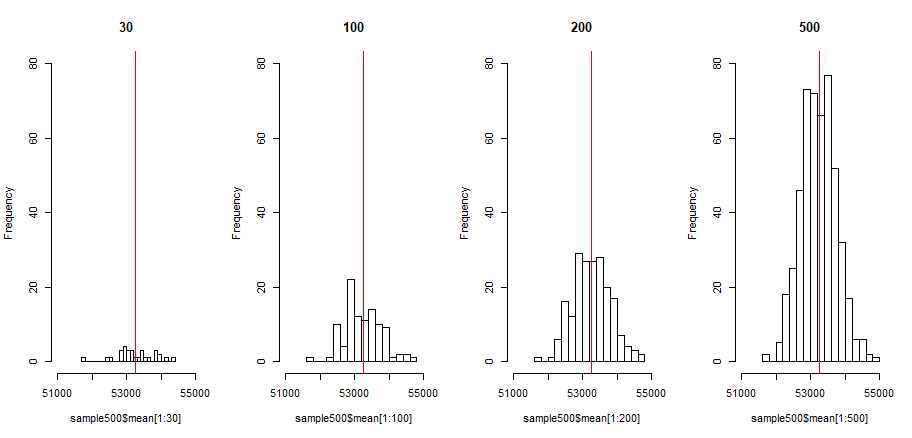# 标准正态分布概率
> ((53253.55+200)-(53253.55-200))/447.9483
 0.8929602

# 计算面积
> pnorm(0.8929602, 0, 1)
 0.8140608


## 4. 区间估计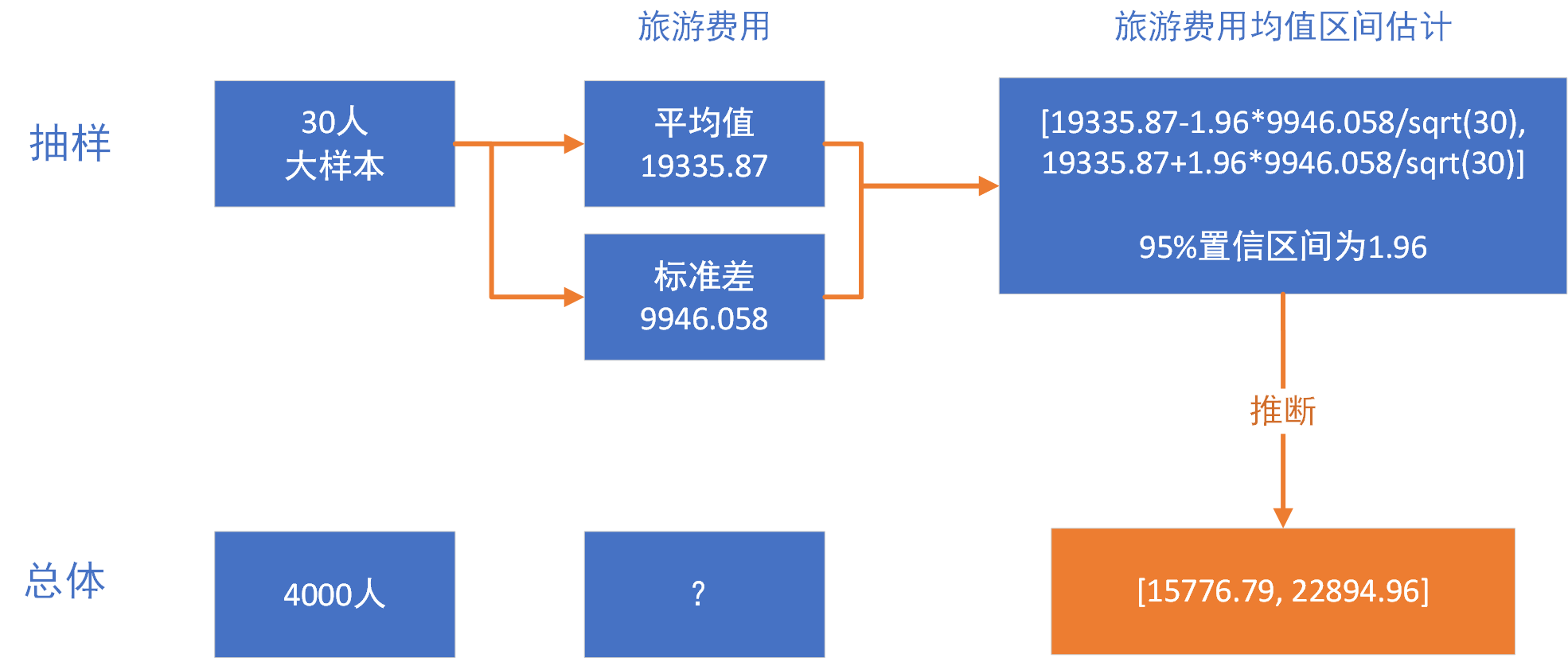> total_m<-20000              # 总体均值
> total_sd<-5000              # 总体标准差
> s<-30                 # 样本数据
> min<-5000             # 样本最小值
> max<-65000            # 样本最大值

# 生成样本数据
> set.seed(10)
> price<-rnorm(s-2,total_m,total_sd)          # 正态分布

# 查看样本数据
> price<-c(min,max,price);price               # 拼接最大值，最小值
  5000.000 65000.000 20093.731 19078.737 13143.347
 17004.161 21472.726 21948.972 13959.619 18181.620
 11866.637 18717.608 25508.898 23778.908 18808.832
 24937.224 23706.951 20446.736 15225.281 19024.248
 24627.606 22414.893 17018.447  9073.566 16625.670
  9404.694 13674.010 18131.692 16562.223 15639.206

# 样本均值
> sample_m<-mean(price);sample_m
 19335.87

# 样本标准差
> sample_sd<-sd(price);sample_sd
 9946.058


# 排序
> price<-price[order(price)]

# 画出散点图
> plot(price,type='both')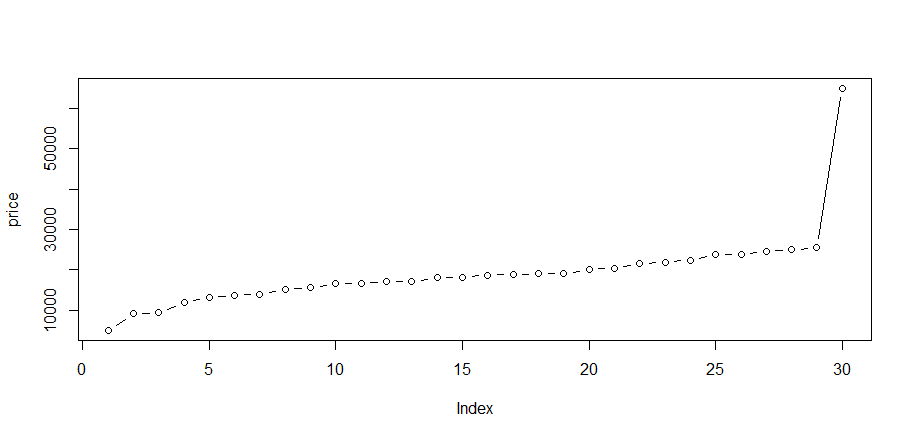• 大样本(>=30)，已知总体方差，对总体均值进行区间估计。
• 大样本(>=30)，未知总体方差，对总体均值进行区间估计。
• 小样本(<30)，总体符合正态分布时，已知总体方差。
• 小样本(<30)，总体符合正态分布时，未知总体方差。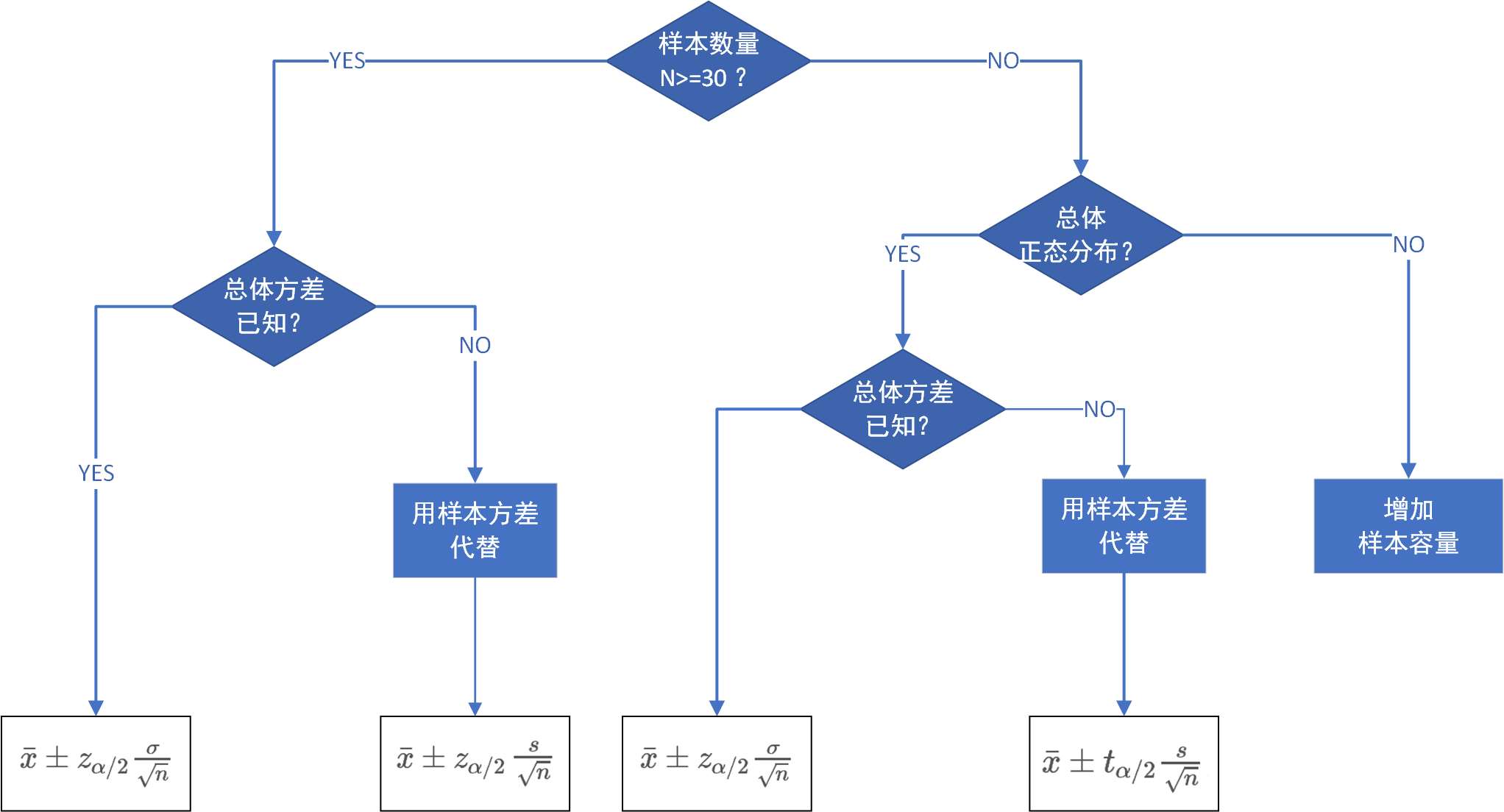• 样本均值: x¯
• 样本数量: n
• 样本方差: s
• 总体方差: σ
• Z分布：z
• T分布:  t
• 显著性水平alpha: α

# 进行区间估计
# 参数为：样本，标准差，置信区间
> conf.int=function(x,sigma,conf.level=0.95) {
+   mean<-mean(x)
+   n<-length(x)
+   alpha<-1-conf.level
+   z=qnorm(1-alpha/2,mean=0,sd=1,lower.tail = T)
+   c(mean-sigma*z/sqrt(n),mean+sigma*z/sqrt(n))
+ }


> conf.int(price,total_sd,0.95)
 17546.68 21125.07


> conf.int(price,sample_sd,0.95)
 15776.79 22894.96


# 安装BSDA包
> install.packages('BSDA')
> library(BSDA)

# 当已知总体标准差时，对总体均值进行区间估计。
> z.test(price,sigma.x=total_sd)
One-sample z-Test
data:  price
z = 21.181, p-value < 2.2e-16
alternative hypothesis: true mean is not equal to 0
95 percent confidence interval:
17546.68 21125.07
sample estimates:
mean of x
19335.87

# 当未知总体标准差时，用样本方标准差代替，对总体均值进行区间估计。
> z.test(price,sigma.x=sd(price))
One-sample z-Test
data:  price
z = 10.648, p-value < 2.2e-16
alternative hypothesis: true mean is not equal to 0
95 percent confidence interval:
15776.79 22894.96
sample estimates:
mean of x
19335.87


> t.test(price)
One Sample t-test
data:  price
t = 10.648, df = 29, p-value = 1.558e-11
alternative hypothesis: true mean is not equal to 0
95 percent confidence interval:
15621.96 23049.79
sample estimates:
mean of x
19335.87